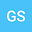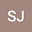loading page

The heat semigroup and equation related to a Bessel-type operators and the canonical Fourier Bessel transform
••Ghazouani SamiUniversity of Carthage, Faculty of Science of Bizerte, (UR17ES21),"Dynamical systems and their applications" 7021, Jarzouna, Bizerte,Tunisia
Author ProfileSahbani Jihed
Faculty of Sciences of Tunis, University of Tunis El Manar, 2092 Tunis, Tunisia
Author Profile## Abstract

In this paper we study a translation operator associated with the canonical Fourier Bessel transform $\mathcal{F}_{\nu}^{\mathbf{m}}.$ We then use it to derive a convolution product and study some of its important properties. As a direct application, we introduce the heat semigroup generated by the Bessel-type operators $$\Delta_{\nu}^{\mathbf{m}^{-1}}=\frac{d^{2}}{dx^{2}}+\left( \frac{2\nu +1}{x}+2i \frac{a}{b} x\right) \frac{d}{dx}-\left( \frac{a^{2}}{b^{2}}x^{2}-2i\left( \nu +1\right) \frac{a}{b}\right)$$ and use it to solve the initial value problem for the heat equation governed by $\Delta_{\nu}^{\mathbf{m}^{-1}}.$

#### Peer review status:UNDER REVIEW

28 May 2021Submitted to Mathematical Methods in the Applied Sciences
30 May 2021Assigned to Editor
30 May 2021Submission Checks Completed
08 Jun 2021Reviewer(s) Assigned